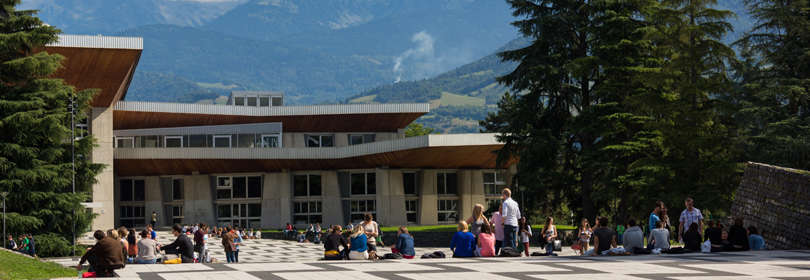Our engineering & Master degrees

School of engineering in Physics, Applied Physics, Electronics & Materials
Science# Numerical methods - 3PMNNUM7

A+Augmenter la taille du texteA-Réduire la taille du texteImprimer le documentEnvoyer cette page par mail cet article Facebook Twitter Linked In
• #### Number of hours

• Lectures : 18.0
• Tutorials : 6.0
• Laboratory works : 0
• Projects : 42.0
• Internship : 0
ECTS : 3.5

### Goals

Acquire basic knowledge in various numerical methods applied to material science

Contact Guillaume PARRY, Annie FOURNIER

Content

Numerical methods for differential equations. Numerical methods for ordinary differential equations : one step methods, multi steps methods, explicit and implicit methods, prediction correction methods. Notions of precision and stability are presented. Numerical methods to solve partial differential equations : finite difference method, finite volume method, finite element method.

Prerequisites

the course of mathematic
Knowledges : derivative of one variable function and of multiple variables functions, Taylor expansion of functions ; operations between matrices, matrix and vector ; linear system of equations and vectorial operators (gradient, divergence, curl).

Tests

Semester 5 - The exam is given in english onlyWritten exam

Semester 5 - This course is given in english only